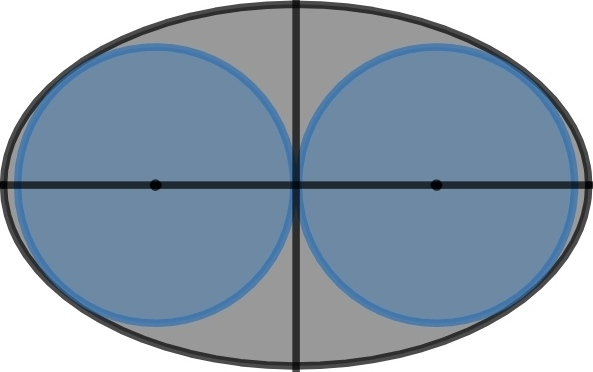# Ellipse's Circular Tangents

Geometry Level 5Inside an ellipse, two circles are drawn which touch the minor axis of the ellipse and also touch the ellipse at the ends of latus rectum. The combined area of the two circles is $A_1$ and area of ellipse is $A_2$.

If $\displaystyle \frac{A_1}{A_2} = \frac{a}{\sqrt{b} + c} \text{ where } a, b, c \in \N \text{ and } \gcd(a,b,c) = 1$, enter answer as $a^2 + b^2 + c^2$.

Try similar problems

All of my problems are original

×